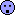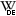## Normal distribution assessment [General Sta­tis­tics]

Hi BE-proff,

» Let's say I have randomly generated set of 1 million values.Before we apply a statistical method, we have to understand thedata generating process.
Therefore: How did you generate your data set? Obtained from RANDOM.ORG? With ahardware random number generator? Software? If yes, whichPRNG? In the last case, most software (even Excel since 2010) implement theMersenne Twis­ter, which is with its period of ≈4.3×106,001 fine for generating large data sets. However, inVBA still anLCG is im­ple­mented, which is bad for large data sets due to its shorter period.

» What criterion should be used to check if the set has normal distribution?Look at the histogram first.set.seed(123456)                                  # for reproducibility x   <- rnorm(1e6, mean = 0, sd = 1)               # or your data instead lim <- c(-max(abs(range(x))), max(abs(range(x)))) # for the plots hist(x, breaks = "FD", freq = FALSE, xlim = lim, col = "bisque", border = NA, las = 1) rug(x, side = 1, ticksize = 0.02) legend("topright", x.intersp = 0,        legend = c(paste("mean(x) =", signif(mean(x), 6)),                   paste("sd(x) =", signif(sd(x), 6))))

Does it look normal? Happy with the mean (should be ≈0) and the standard deviation (should be ≈1)?
If in doubt, overlay it with akernel density estimate.

lines(density(x, n = 2^10), lwd = 3, col = "#FF000080")

Does it match? Not sure? Overlay the normal distribution.

curve(dnorm, lim, lim, n = 2^10, lwd = 2, col = "#0000FF80", add = TRUE)

Still in doubt?

plot(lim, lim, type = "n", xlab = "Theoretical Quantiles",      ylab = "Sample Quantiles", main = "Normal Q-Q Plot", las = 1) grid() qq <- qqnorm(x, plot.it = FALSE) points(qq$x, qq$y, pch = 21, cex = 1.25, col = "#87CEFA80", bg = "#87CEFA80") # patience... qqline(x)

If you insist in a test comparing the data’sempirical cumulative distribution function to the cumulative distribution function of the standard normal:

ks.test(x, "pnorm" , alternative = "two.sided")

Dif-tor heh smusma 🖖Helmut SchützThe quality of responses received is directly proportional to the quality of the question asked. 🚮
Science Quotes22,108 posts in 4,630 threads, 1,567 registered users;
online 4 (0 registered, 4 guests [including 3 identified bots]).
Forum time: Friday 18:34 CEST (Europe/Vienna)

We absolutely must leave room for doubt
or there is no progress and no learning.
There is no learning without having to pose a question.
And a question requires doubt.
People search for certainty.
But there is no certainty.    Richard Feynman

The Bioequivalence and Bioavailability Forum is hosted byIng. Helmut Schütz